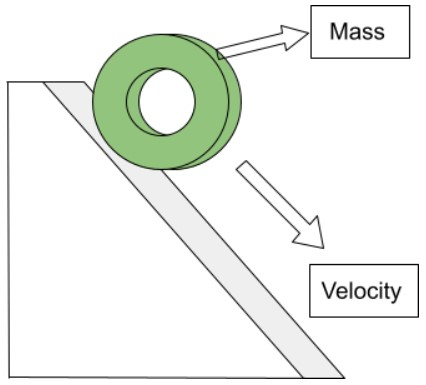# Kinetic Energy Calculator

Input the values of mass and velocity of the object in the kinetic energy calculator to calculate kinetic energy.

kg
m/s

Give Us Feedback

This Kinetic energy calculator can find the energy stored in a body due to its motion, using the mass and velocity of the body. It can also find mass and velocity if the other values are inputted.

## What is Kinetic Energy?

“It is the energy of motion. An object that has motion - whether it is vertical or horizontal motion - has kinetic energy.”

It is defined by a relation between mass and velocity.SI unit of Kinetic energy is the Joule (J). It is because kinetic energy is the work that is required to accelerate a body to its current velocity.

Some types of kinetic energy are:

• Transitional
• Vibrational
• Rotational

## Kinetic energy formula

The kinetic energy equation can be expressed as,

K.E   = (mv2)/2

or

K.E   = 0.5 * m * v * v

Use our potential energy calculator if you need to find P.E of an object.

## How to calculate kinetic energy?

Kinetic energy is very easy to calculate. Multiply the mass to the square of the velocity and divide it by 2. To make it more clear, have a look at the example below:

Example

Calculate the kinetic energy of a wheel of mass 5 kg rolling down from a long slide with a velocity of 20 ms-1.

Solution

Step 1: Write the given information.

Mass =  5 kg

Velocity = 20 ms-1

Step 2: Put in the kinetic energy formula.

K.E = 0.5 * 5 * 20 * 20

K.E = 1000 J

### Math Tools